# PSEB 5th Class Maths Solutions Chapter 5 Money (Currency) Ex 5.4

Punjab State Board PSEB 5th Class Maths Book Solutions Chapter 5 Money (Currency) Ex 5.4 Textbook Exercise Questions and Answers.

## PSEB Solutions for Class 5 Maths Chapter 5 Money (Currency) Ex 5.4

1. Find the value of the following :

Question 1.
₹ 258 × 17
Solution: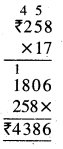Question 2.
₹ 410 × 20
Solution: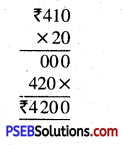Question 3.
₹ 518 × 18
Solution: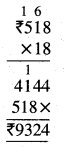Question 4.
₹ 220 × 14
Solution: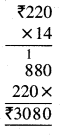Question 5.
₹ 206 × 25.
Solution: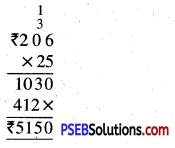2. Evaluate :

Question 1.
₹ 3120 ÷ 10
Solution:
₹ 3120 ÷ 10 = ₹ 312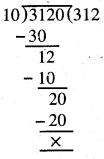Question 2.
₹ 1590 ÷ 15
Solution:
₹ 1590 ÷ 15 = ₹ 106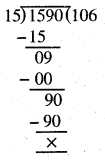Question 3.
₹ 4272 ÷ 16
Solution:
₹ 4272 ÷ 16 = ₹ 267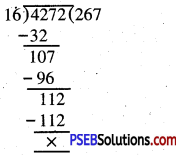Question 4.
₹ 4200 ÷ 20
Solution:
₹ 4200 ÷ 20 = ₹ 210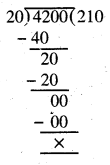Question 5.
₹ 6500 ÷ 25.
Solution:
₹ 6500 ÷ 25 = ₹ 260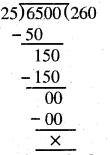Question 3.
The cost of a calculator is ₹ 415. Find the cost of 17 such calculators.
Solution:
The cost price of 1 calculator = ₹ 415
The cost price of 17 calculators = ₹ 415 × 17 = ₹ 7055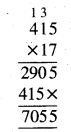The cost price of 17 calculators = ₹ 7055

Question 4.
The price of 1 kg ghee is ₹ 435. What is the price of 18 kg ghee ?
Solution:
The cost price of 1 kg ghee = ₹ 435
The cost price of 18 kg ghee = ₹ 435 × 18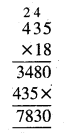Thus, the cost price of 18 kg ghee = ₹ 7830

Question 5.
The cost of 24 glasses is ? 2880. Find the cost of a glass.
Sol. The cost price of 24 glasses = ₹ 2880
The cost price of 1 glass = ₹ 2880 ÷ 24 = ₹ 120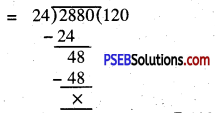The cost price of 1 glass = ₹ 120

Question 6.
The cost of 19 geometry boxes is ₹ 2850. Find the cost of a geometry box.
Solution:
The cost price of 19 geometry boxes = ₹ 2850
The cost price of 1 geometry box = ₹ 2850 ÷ 19 = ₹ 150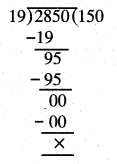The cost price of 1 geometry box = ₹ 1150Question 7.
The price of 1 litre petrol is ₹ 73. What is the cost of 12 litres petrol.
Solution:
Cost of 1 litre of petrol = ₹ 73
Cost of 12 litres of petrol = ₹ 73 × 12 = ₹ 876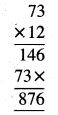Cost of 12 litres of petrol = ₹ 876

Question 8.
The cost of 25 kg rice is ₹ 2000. Find the cost of 1 kg rice.
Solution:
Cost of 25 kg of rice = ₹ 2000
Cost of 1 kg of rice = ₹ 2000 ÷ 25 = ₹ 80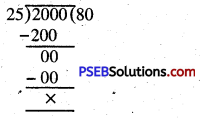Cost of 1 kg of rice = ₹ 80

Question 9.
The price of 1 m cloth is ₹ 500. Find the cost of 18 m cloth.
Solution:
Cost of 1 m cloth = ₹ 500
Cost of 18 m cloth = ₹ 500 × 18 = ₹ 9000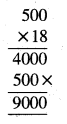Cost of 18 m cloth = ₹ 900010. Fill in the blanks :

Question 1.
₹ 13 × 8 = ____________
Solution:
₹ 104

Question 2.
₹ 24 × 5 = ___________
Solution:
₹ 120

Question 3.
₹ 24 ÷ 3 = __________
Solution:
₹ 8Question 4.
₹ 72 ÷ 8 = ____________
Solution:
₹ 9

Question 5.
₹ 25 × 6 = __________
Solution:
₹ 150

Question 6.
₹ 100 ÷ 10 = __________
Solution:
₹ 10

Question 7.
There are __________ ₹ 100 notes in ₹ 1000.
Solution:
10Question 8.
There are ___________ ₹ 50 notes in ₹ 300.
Solution:
6

Question 9.
There are ___________ ₹ 20 notes in ₹ 500.
Solution:
25

Question 10.
There are _________ ₹ 500 notes in ₹ 2000.
Solution:
4